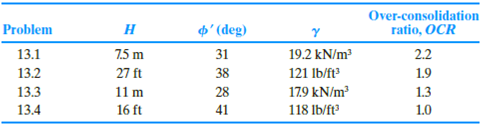Chapter 13, Problem 13.4PPrinciples of Geotechnical Enginee...

9th Edition
Braja M. Das + 1 other
ISBN: 9781305970939

Solutions

Chapter
SectionPrinciples of Geotechnical Enginee...

9th Edition
Braja M. Das + 1 other
ISBN: 9781305970939
Textbook Problem

13.1 through 13.4 Figure 13.39 shows a retaining wall that is restrained from yielding. For each problem, determine the magnitude of the lateral earth force per unit length of the wall. Also, find the location of the resultant, z ¯ , measured from the bottom of the wall.Figure 13.39

To determine

Find the magnitude of the lateral earth forces Po per unit length of the wall and find the location z¯ of the resultant measured from the bottom of the wall.

Explanation

Given information:

The height (H) of the retaining wall is 16 ft.

The soil friction angle ϕ is 41°.

The angle of wall friction δ is 0.

The unit weight γ of the sand is 118lb/ft3

The over-consolidation ratio (OCR) is 1.0.

Calculation:

Calculate the earth pressure coefficient (Ko) using the relation.

Ko=(1sinϕ)(OCR)sinϕ

Substitute 41° for ϕ and 1.0 for OCR.

Ko=(1sin41°)(1.0)sin41°=0.34394×1.0=0.344

Calculate the total force per unit length (Po) using the relation.

Po=12KoγH2

Substitute 0

Still sussing out bartleby?

Check out a sample textbook solution.

See a sample solution

The Solution to Your Study Problems

Bartleby provides explanations to thousands of textbook problems written by our experts, many with advanced degrees!

Get Started

Define each of the following terms: a. data b. field c. record d. file

Database Systems: Design, Implementation, & Management

What system is the predecessor of almost all modern multiuser systems?

Principles of Information Security (MindTap Course List)

What is risk management?

Management Of Information Security

Describe the motion of the electrode in keyhole welding.

Welding: Principles and Applications (MindTap Course List)

Define the terms, magnetic stripe card and smart card. Describe the uses of each.

Enhanced Discovering Computers 2017 (Shelly Cashman Series) (MindTap Course List)